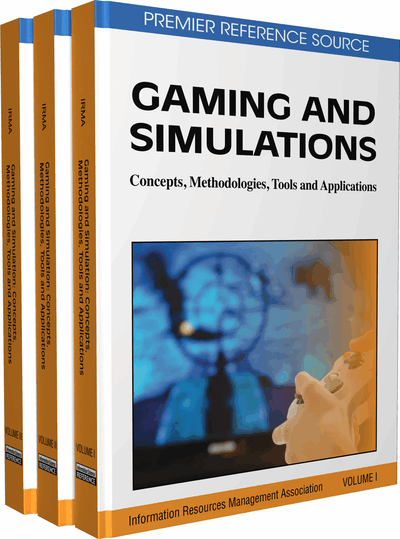# Computer Simulations and Scientific Knowledge Construction

Athanassios Jimoyiannis
DOI: 10.4018/978-1-60960-195-9.ch104
OnDemand:
(Individual Chapters)
Available
\$37.50
No Current Special Offers

## Abstract

In this article, the basic characteristics of scientific and educational simulations are discussed. Research findings which support their educational effectiveness are presented, and emphasis is placed on the pedagogical issues of designing and using simulation environments aiming at facilitating students’ engagement and active knowledge construction.
Chapter Preview
Top

## Scientific Simulations

Generally speaking, a simulation is a technique of imitating the behaviour of a situation, process, or system by means of an analogous system. In the simplest sense, a system is a set of interacting identities. In the case of scientific simulations, this analogous system is a mathematical model. The mathematical equations that produce the model represent the various processes which take place within the target system. In other words, this model constitutes a simplified or idealised representation of a system by means of a set of mathematical equations (algebraic, differential, or integral). The mathematical model becomes a simulation by solving numerically (i.e., for varying sets of input values) the equations comprising in order to imitate or simulate the dynamic (time-varying) behaviour of the system (Fishwick, 1995).

In a computer simulation, the mathematical model is produced by proper executable algorithms, which are used to solve the mathematical equations. Consequently, a computer-based simulation is a software application that embodies a model of the actual or theoretical system, executing the model on a computer and analysing the output. Any system in either the micro- or the macro-world can be simulated, providing that its behaviour can be described by a computer model (algorithm). Usually, a simulation model is an abstraction that behaves somewhat like the original system, thus allowing users to replicate only a small part of the actual system under investigation (e.g., its key features or characteristics).

## Complete Chapter List

Search this Book:
Reset